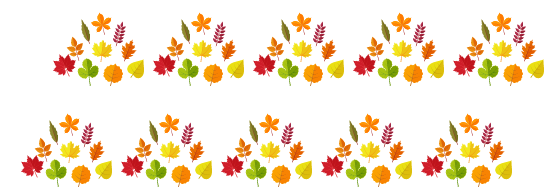Mathematics
Easy

Question

# Count the leaves by 10s.## 501003080Hint:

## The correct answer is: 100

### Here, we  have to count the leaves by 10s.Count by 10s = 10, 20, 30, 40, 50, 60, 70, 80, 90,100. 10 tens are 100 . So, there are 100 leaves.Hence, the correct option is (a).

Counting is the act of determining the quantity or the total number of objects in a set or a group.

### Related Questions to study#### With Turito Foundation.#### Get an Expert Advice From Turito.